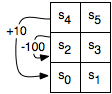Practice Exercise 11.B
SARSA

## 2: Learning Goals

• Use the SARSA algorithm to generate the table Q[S,A].
• Compare SARSA to the Q-learning algorithm.
• Modify parameters to enhance the performance of SARSA.

## 3: Directed Questions

1. Given a set of states S, a set of actions A, and an experience ⟨s,a,r,s',a'⟩, what is the time complexity to update the value of Q(s,a) using SARSA? [solution]

2. Consider the following Q[S,A] table:

State 1 State 2 1.5 2.5 4 3

Assume that α=0.1, and γ=0.5. After the experience ⟨1,1,5,2,1⟩, which value of the table gets updated and what is its new value? [solution]

3. What is one major difference between an off-policy learner and an on-policy learner? [solution]

4. Describe one possible scenario in which SARSA would use a different policy than Q-learning. [solution]

## 4: Exercise: David's Tiny Game

Consider the tiny reinforcement learning problem shown in the following figure:This problem is also discussed in Section 11.3 of the textbook. There are six states the agent could be in, labeled as s0,...,s5. The agent has four actions: UpC, Up, Left, Right. That is all the agent knows before it starts. It does not know how the states are configured, what the actions do, or how rewards are earned. Suppose the actions work as follows:
upC
(for "up carefully") The agent goes up, except in states s4 and s5, where the agent stays still, and has a reward of -1.
right
The agent moves to the right in states s0,s2,s4 with a reward of 0 and stays still in the other states, with a reward of -1.
left
The agent moves one state to the left in states s1,s3,s5. In state s0, it stays in state s0 and has a reward of -1. In state s2, it has a reward of -100 and stays in state s2. In state s4, it gets a reward of 10 and moves to state s0.
up
With a probability of 0.8 it acts like upC, except the reward is 0. With probability 0.1 it acts as a left, and with probability 0.1 it acts as right.
The following applet can be used to simulate Q-learning and SARSA for this problem:

Visit this page for instructions on how to use the applet. Click the "use SARSA" checkbox to switch between Q-learning and SARSA. Consider the following parameter settings:
1. SARSA, α=0.1, γ=0.5, Greedy Exploit = 80%, and initial Q-value = 0.
2. Q-learning, α=0.1, γ=0.5, Greedy Exploit = 80%, and initial Q-value = 0.
3. SARSA, α=0.1, γ=0.5, Greedy Exploit = 100%, and initial Q-value = 20.
4. Q-learning, α=0.1, γ=0.5, Greedy Exploit = 100%, and initial Q-value = 20.
5. SARSA, α=0.1, γ=0.1, Greedy Exploit = 80%, and initial Q-value = 0.
6. SARSA, α=0.9, γ=0.5, Greedy Exploit = 80%, and initial Q-value = 0.
Run an experiment for each of these parameter settings and record the total reward received. [solution] Use a step size of 1,000,000. Manually move the robot to the bottom left square and press the reset button before each experiment. Keep in mind that since there is randomization involved, there will be some variation when running the same experiment multiple times.
• Compare the results of the first experiment to the second experiment. One should do significantly better than the other. Explain why changing the learning algorithm causes this result. [solution]

• Run the first and second experiment multiple times and inspect the resulting policies (indicated by the blue arrows). What is the major difference between policies generated by SARSA and Q-learning? [solution]

• Compare the results of the first and second experiments to the third and fourth experiments. Changing the learning algorithm should have a much larger effect on one of these experimental pairs. Explain why these parameter settings cause this result. [solution]

• Compare the results of the first experiment to the fifth experiment. One should do significantly better than the other. Explain why the change in the γ parameter causes this result. [solution]

• Compare the results of the first experiment to the sixth experiment. One should do significantly better than the other. Explain why the change in the α parameter causes this result. [solution]

## 5: Learning Goals Revisited

• Use the SARSA algorithm to generate the table Q[S,A].
• Compare SARSA to the Q-learning algorithm.
• Modify parameters to enhance the performance of SARSA.